# Lesson 12: Atoms

12.1 What are atoms? – 12.2 Line spectra – 12.3 Bohr’s model of the atom – 12.4 Farewell to Bohr’s atomic model
12.5 Quantization of energy in the Franck-Hertz experiment – 12.6 Three-dimensional potential well and orbitals – 12.7 Progress check – 12.8 Summary

We have so far considered only quantum objects such as electrons or photons. This chapter will turn its attention to atoms. The focus here is on Bohr’s model of the atom, the quantization of energy, and the three-dimensional potential well.

### 12.1 What are atoms?

The name atom (= indivisible) originates from a time when atoms were still considered to be the smallest building blocks of matter.

Modern physics has shown, however, that atoms consist of a very small, positively charged atomic nucleus and electrons bound to it. They are therefore not elementary building blocks.

### 12.2 Line spectra

If you observe the light from different fluorescent tubes or a mercury vapor lamp with the aid of a spectroscope, you will see only individual lines in specific colors instead of the usual continuous spectrum of an incandescent lamp, for example (Experiment 12.1). Such a spectrum is called a discrete or line spectrum.In the 19th century, it was not possible to explain these spectra. The efforts to find an explanation were a trigger for the development of quantum mechanics.
In 1885, Balmer found a mathematical relationship on the basis of the lines observed for atomic hydrogen, which predicted further, hitherto unobserved lines:

The spectral lines of atomic hydrogen are described by the following expression (Balmer formula):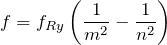where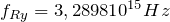is a constant, the so-called Rydberg frequency. The number m classifies the different series of hydrogen: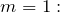Lyman series in the ultraviolet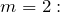Balmer series partially in the visible region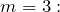Paschen series in the infrared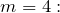Brackett series in the infrared.

n is a natural number (with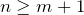) , which identifies the lines within a series.

### 12.3 Bohr’s model of the atom

In 1913, Niels Bohr proposed a model of the atom which still serves as a popular concept. This model by Bohr was a very important intermediate step on the road to today’s understanding of atoms and it enabled a line spectrum to be explained for the first time. Some of its assumptions have remained valid until this day:

The angular momentum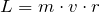is a multiple of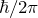. The energy in atoms is therefore quantized as well. The electrons in the atom can exist only in states whose energies have very specific values (the so-called stationary states).

An atom only emits a photon when an electron moves from a stationary state with energy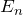into another state with a lower energy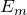. The frequency of the emitted photon is given by: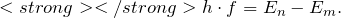### 12.4 Farewell to Bohr’s atomic model

With the development of quantum theory, it turned out that Bohr’s model of the atom with the assumptions of 1913 was not correct. Bohr’s model already meant a break with the laws of classical physics (ad hoc postulate of only certain permissible orbits, non-radiative orbit). It turned out that even more fundamental modifications were necessary, e. g.

• The electrons do not circle on well-defined orbits around the nucleus, i.e. they cannot be ascribed a position (cf. double-slit experiments in Chapter 6).

This statement can be justified with Heisenberg’s uncertainty relation, which states that the average kinetic energy of electrons localized on orbits exceeds the energy of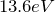required for ionization many times over. The atom would be ionized immediately and thus destroyed.

Is Bohr’s model of the atom still up-to-date?

### 12.5 Quantization of energy in the Franck-Hertz experiment

One experiment which supports Bohr’s ad hoc formulation of the quantization of energy is the Franck-Hertz experiment (Experiment 12.3). The experiment shows that mercury atoms can only absorb a very specific amount of energy.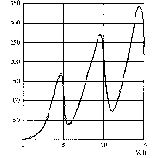### 12.6 Three-dimensional potential well and orbitals

In quantum mechanics, the quantization of the energy does not need to be postulated, as is the case with Bohr’s model; instead it emerges naturally from the theory (cf. Chapter 10).

An analogy can be found between an electron in the hydrogen atom and an electron in a potential well: Both electrons are “confined”, i. e. they are bound to a certain spatial region by forces.

In the first approximation we can therefore consider the infinite potential well as a “model atom”, but now in three-dimensional space.

The one-dimensional Schrödinger equation for the infinitely high potential well is: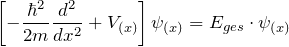The extension to the three-dimensional case is quite simple: Since all three coordinates are equal, the following applies:. The kinetic energy operator is therefore: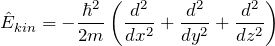and the Schrödinger equation is: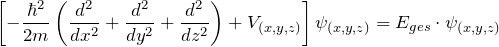Since the potential in the interior of the well is constant, the expedient approach to a solution is the product of the solutions for one-dimensional potential wells: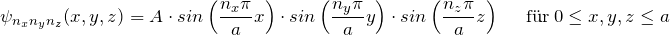Inserting this approach into the Schrödinger equation yields: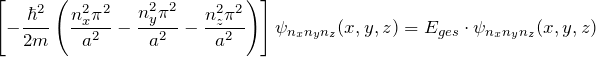With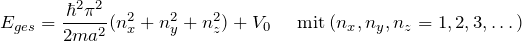the Schrödinger equation is fulfilled.

We have thus found a wave function and the possible energies for the three-dimensional potential well.

Interpretation:

Instead of one quantum number, three quantum numbers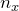,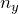and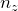now appear. Since these three numbers can independently have all possible values, this results in a large number of possibilities. In order to illustrate the transitions graphically, the energy levels are entered into an energy level diagram.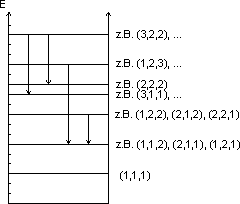The ground state is given by the quantum number combination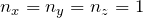. Figure (a) shows its probability density.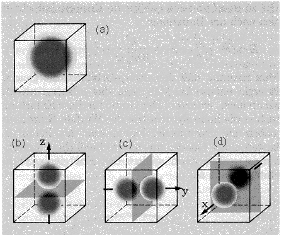There are three states for the next highest energy eigenvalue, which are given by the quantum number combinations

*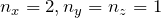(Figure (b))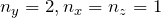(Figure (c))
*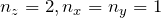(Figure (d))

All three states (b) – (d) have the same energy.

The probability distributions can be illustrated for different values of the quantum numbers. They correspond to the orbitals familiar from chemistry. They are used to describe the spatial regions in which the probability of finding an electron is particularly high.

Probability density for antiprotonic hydrogen.

### 12.7 Progress check

The following points were important in this chapter:

Line spectrum of glowing gases.
Bohr’s model of the atom (energy quantization, frequency of the emitted photons).
What has to be revised in respect of Bohr’s model of the atom?
Franck-Hertz experiment.
Formulation of the problem and solution of the three-dimensional potential well with the Schrödinger equation.
Before you move on to the next chapter, make sure you know the fundamental ideas behind these points. You can then check this with the aid of the Summary.

### 12.8 Summary of Chapter 12: Atoms

In addition to the continuous spectra, line spectra (or also discrete spectra) can be observed, e. g. for glowing gases. These can only be explained with the aid of quantum mechanics.

The statements formulated by Bohr in his atomic model and still applicable today are:

• The quantization of energy, which is also supported by the Franck-Hertz experiment,
• The frequency of the photons emitted in the transition from the energy stateinis: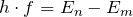The concept of well-defined electron orbits around the atomic nucleus cannot be upheld, since it contradicts Heisenberg’s uncertainty relation.

The simple potential well discussed in Chapter 10 is extended to three dimensions. This model of the three-dimensional potential well can be seen as a precursor of the atom. A natural explanation for the existence of atomic orbitals results from the treatment of the potential well.